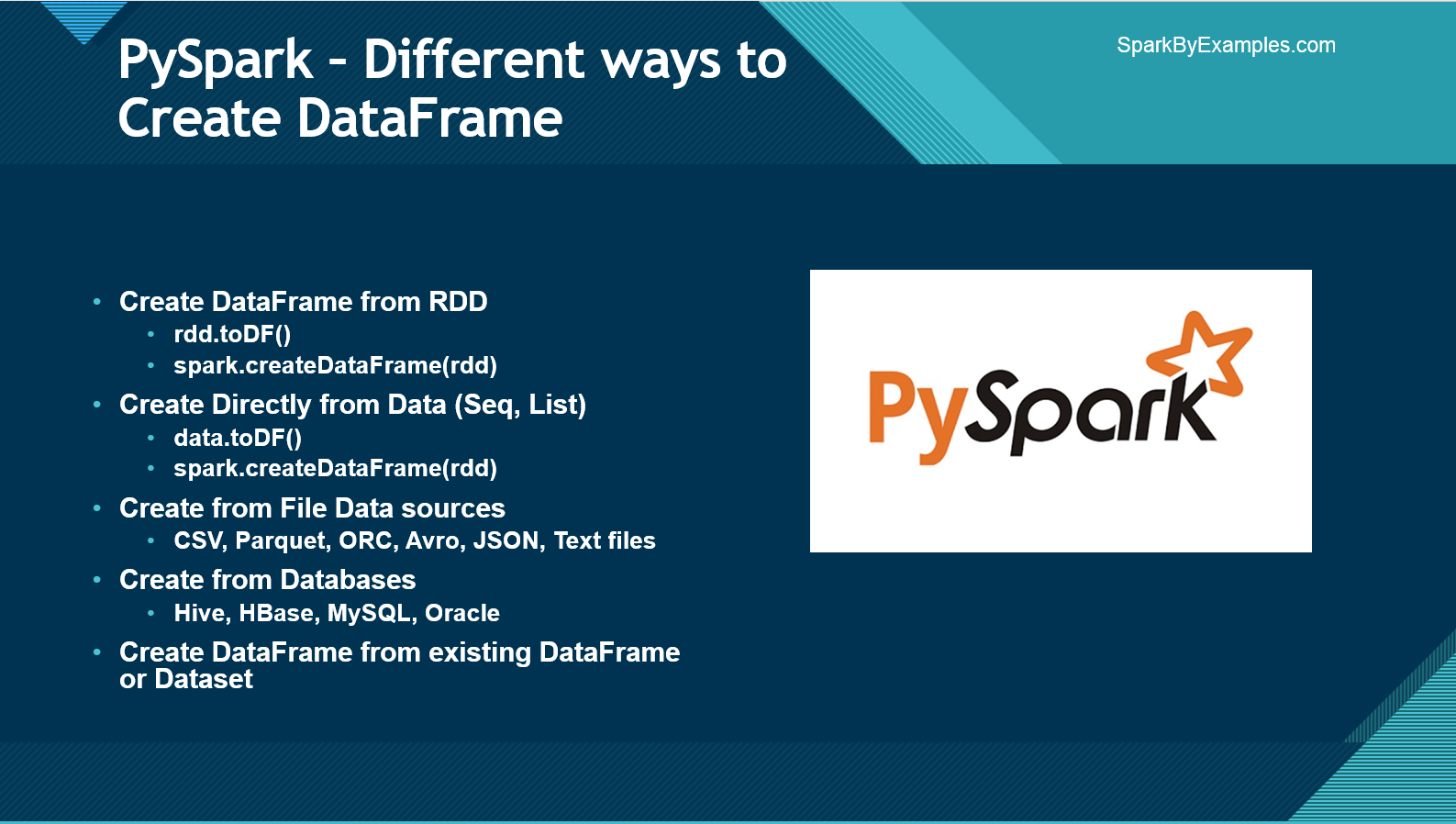# PySpark – Create DataFrame with Examples

You can manually create a PySpark DataFrame using `toDF()` and `createDataFrame()` methods, both these function takes different signatures in order to create DataFrame from existing RDD, list, and DataFrame.

You can also create PySpark DataFrame from data sources like TXT, CSV, JSON, ORV, Avro, Parquet, XML formats by reading from HDFS, S3, DBFS, Azure Blob file systems e.t.c.

Related:

Finally, PySpark DataFrame also can be created by reading data from RDBMS Databases and NoSQL databases.

In this article, you will learn to create DataFrame by some of these methods with PySpark examples.

In order to create a DataFrame from a list we need the data hence, first, let’s create the data and the columns that are needed.

``````
columns = ["language","users_count"]
data = [("Java", "20000"), ("Python", "100000"), ("Scala", "3000")]
``````

## 1. Create DataFrame from RDD

One easy way to manually create PySpark DataFrame is from an existing RDD. first, let’s create a Spark RDD from a collection List by calling parallelize() function from SparkContext . We would need this rdd object for all our examples below.

``````
spark = SparkSession.builder.appName('SparkByExamples.com').getOrCreate()
rdd = spark.sparkContext.parallelize(data)
``````

### 1.1 Using toDF() function

PySpark RDD’s toDF() method is used to create a DataFrame from the existing RDD. Since RDD doesn’t have columns, the DataFrame is created with default column names “_1” and “_2” as we have two columns.

``````
dfFromRDD1 = rdd.toDF()
dfFromRDD1.printSchema()
``````
``````
root
|-- _1: string (nullable = true)
|-- _2: string (nullable = true)
``````

If you wanted to provide column names to the DataFrame use `toDF() `method with column names as arguments as shown below.

``````
columns = ["language","users_count"]
dfFromRDD1 = rdd.toDF(columns)
dfFromRDD1.printSchema()
``````

This yields the schema of the DataFrame with column names. use the show() method on PySpark DataFrame to show the DataFrame

``````
root
|-- language: string (nullable = true)
|-- users: string (nullable = true)
``````

By default, the datatype of these columns infers to the type of data. We can change this behavior by supplying schema, where we can specify a column name, data type, and nullable for each field/column.

### 1.2 Using createDataFrame() from SparkSession

Using createDataFrame() from SparkSession is another way to create manually and it takes rdd object as an argument. and chain with toDF() to specify name to the columns.

``````
dfFromRDD2 = spark.createDataFrame(rdd).toDF(*columns)
``````

## 2. Create DataFrame from List Collection

In this section, we will see how to create PySpark DataFrame from a list. These examples would be similar to what we have seen in the above section with RDD, but we use the list data object instead of “rdd” object to create DataFrame.

### 2.1 Using createDataFrame() from SparkSession

Calling `createDataFrame()` from `SparkSession `is another way to create PySpark DataFrame manually, it takes a list object as an argument. and chain with `toDF()` to specify names to the columns.

``````
dfFromData2 = spark.createDataFrame(data).toDF(*columns)
``````

### 2.2 Using createDataFrame() with the Row type

`createDataFrame()` has another signature in PySpark which takes the collection of Row type and schema for column names as arguments. To use this first we need to convert our “data” object from the list to list of Row.

``````
rowData = map(lambda x: Row(*x), data)
dfFromData3 = spark.createDataFrame(rowData,columns)
``````

### 2.3 Create DataFrame with schema

If you wanted to specify the column names along with their data types, you should create the StructType schema first and then assign this while creating a DataFrame.

``````
from pyspark.sql.types import StructType,StructField, StringType, IntegerType
data2 = [("James","","Smith","36636","M",3000),
("Michael","Rose","","40288","M",4000),
("Robert","","Williams","42114","M",4000),
("Maria","Anne","Jones","39192","F",4000),
("Jen","Mary","Brown","","F",-1)
]

schema = StructType([ \
StructField("firstname",StringType(),True), \
StructField("middlename",StringType(),True), \
StructField("lastname",StringType(),True), \
StructField("id", StringType(), True), \
StructField("gender", StringType(), True), \
StructField("salary", IntegerType(), True) \
])

df = spark.createDataFrame(data=data2,schema=schema)
df.printSchema()
df.show(truncate=False)
``````

This yields below output.

## 3. Create DataFrame from Data sources

In real-time mostly you create DataFrame from data source files like CSV, Text, JSON, XML e.t.c.

PySpark by default supports many data formats out of the box without importing any libraries and to create DataFrame you need to use the appropriate method available in `DataFrameReader` class.

### 3.1 Creating DataFrame from CSV

Use `csv()` method of the `DataFrameReader` object to create a DataFrame from CSV file. you can also provide options like what delimiter to use, whether you have quoted data, date formats, infer schema, and many more. Please refer PySpark Read CSV into DataFrame

``````
``````

### 3.2. Creating from text (TXT) file

Similarly you can also create a DataFrame by reading a from Text file, use `text()` method of the DataFrameReader to do so.

``````
``````

### 3.3. Creating from JSON file

PySpark is also used to process semi-structured data files like JSON format. you can use `json()` method of the DataFrameReader to read JSON file into DataFrame. Below is a simple example.

``````
``````

Similarly, we can create DataFrame in PySpark from most of the relational databases which I’ve not covered here and I will leave this to you to explore.

## 4. Other sources (Avro, Parquet, ORC, Kafka)

We can also create DataFrame by reading Avro, Parquet, ORC, Binary files and accessing Hive and HBase table, and also reading data from Kafka which I’ve explained in the below articles, I would recommend reading these when you have time.

Happy Learning !!

### NNK

SparkByExamples.com is a Big Data and Spark examples community page, all examples are simple and easy to understand and well tested in our development environment Read more ..

### This Post Has 4 Comments

1.Anonymous

What is significance of * in below
dfFromData2 = spark.createDataFrame(data).toDF(*columns

2.Anonymous

* indicates: its passing list as an argument

3.Anonymous

regular expression for arbitrary column names

4.sagar

What is significance of * in below
dfFromData2 = spark.createDataFrame(data).toDF(*columns)